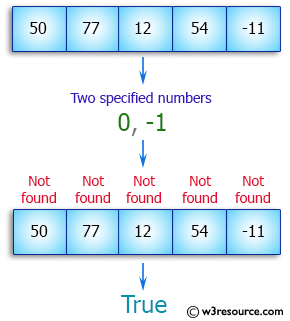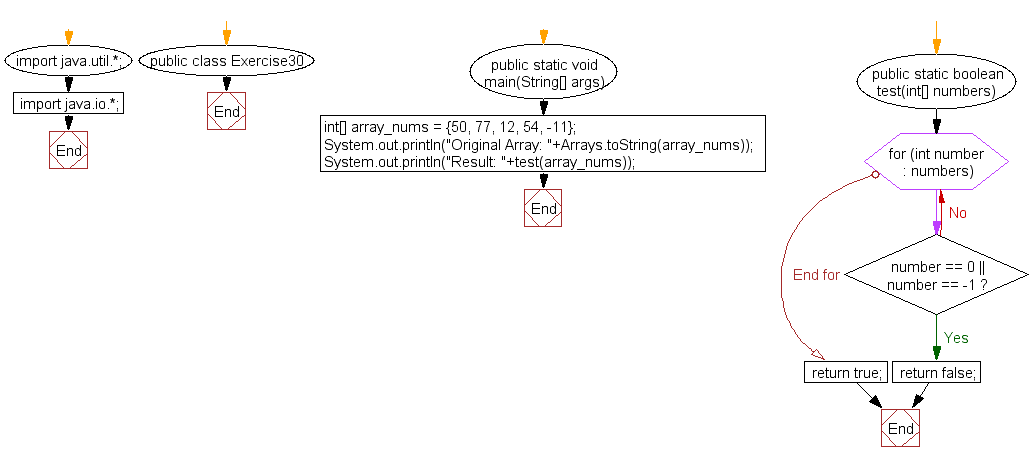﻿ Java exercises: Check if an array of integers two specified numbers - w3resource# Java Array Exercises: Check if an array of integers without two specified numbers

## Java Array: Exercise-30 with Solution

Write a Java program to check if an array of integers without 0 and -1.

Pictorial Presentation:Sample Solution:

Java Code:

``````import java.util.*;
import java.io.*;
public class Exercise30 {
public static void main(String[] args)
{
int[] array_nums = {50, 77, 12, 54, -11};
System.out.println("Original Array: "+Arrays.toString(array_nums));
System.out.println("Result: "+test(array_nums));
}
public static boolean test(int[] numbers) {
for (int number : numbers) {
if (number == 0 || number == -1) {
return false;
}
}
return true;
}
}
```
```

Sample Output:

```
Original Array: [50, 77, 12, 54, -11]
Result: true
```

Flowchart:Visualize Java code execution (Python Tutor):

Java Code Editor:

Improve this sample solution and post your code through Disqus

What is the difficulty level of this exercise?

﻿

## Java: Tips of the Day

Choice between Float and Double:

Data type Bytes used Significant figures (decimal)
Float 4 7
Double 8 15

Double is often preferred over float in software where precision is important because of the following reasons:
Most processors take nearly the same amount of processing time to perform operations on Float and Double. Double offers far more precision in the same amount of computation time.

Ref: https://bit.ly/3oj7K2K